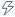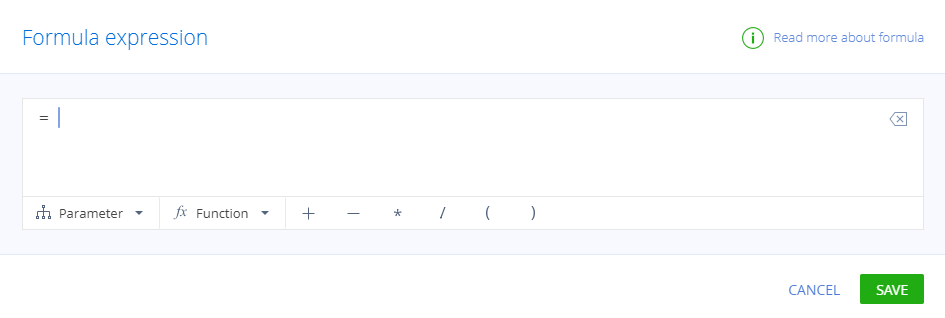# Formulas in business logic and pivot tables

Products
All Creatio products

Save time your employees spend on data processing by automatically calculating dates and numeric values in section pages and pivot tables. For instance, you can calculate a product price after taxes, an ETA for a task or a contract amendment date. To set up these calculations, use formulas in the “Set field value” business rule and in pivot table settings. You can navigate to the formula setup window:

• by clickingwhen setting up the Set field value business rule;
• by clicking Add calculated field in the pivot table settings.

This will open the formula setup window (Fig. 1).

Fig. 1 Formula setup window• Constants and variables. For example, you can enter any number or you can use the page field values from the formula setup window's Parameter menu.
• Mathematical operators (+, –, *, /, brackets).
• There also are several function groups used with dates: Date difference, Add to date, Date part, CurrentDateTime. The function by itself is a valid formula, but you can also combine functions with other elements.

## Date calculation functions for formulas

Function group Description Function Pivot tables Business Rules
Add to date Adds the specified number of years/weeks/hours, etc. to the date. The calculation result's data type is “Date/Time”. AddYear + +
Date difference Calculates how many years/weeks/hours, etc. there are between the dates. The calculation result's data type is “Integer”.
Creatio uses calendar boundaries to calculate the difference. For instance, DiffYear(2020-12-31, 2021-01-01) = 1
If the first date in the function is later than the second date, the calculation result will be negative.
DiffYear + +
DiffQuarter +
DiffMonth + +
DiffWeek +
DiffDay + +
DiffHour + +
DiffMinute + +
Date part

Determines the number of the original date's year/month/day, etc. The function always uses the 24-hour format. For instance, the date 02.16.2021 3:38 PM contains the following:

• 2021th year,
• 2nd month,
• 8th week,
• 3rd day of the week,
• 16th day,
• 15th hour.
The calculation result has an “Integer” data type.
PartYear +
PartMonth +
PartWeek +
PartDay +
PartDayWeek +
PartHour +
CurrentDateTime Determines the current date and time. The calculation result's data type is “Date/Time”. The function's brackets have to remain empty. CurrentDateTime +

Example. Calculate the sum of per diem payments to issue an employee.
```Target field: [Sum to issue]
Variables: the values of fields [Per diem] and [Duration, days].
Formula: = [Per diem] * [Duration, days]```
Example. Calculate the call duration.
```Target field: [Duration]
Elements: the values of fields [Start date] and [End date].
Formula: = DiffMinute([End date], [Start date])```
Example. Calculate how many years an employee has worked for the company.
```Target field: [Works for the company, years]
Elements: the values of [Career start date] field and the current date.
Formula: = DiffYear(CurrentDateTime(), [Career start date])```
Example: Calculate support agent's efficiency
```Target field: [Efficiency, %]
Elements: [Cases closed during the month], [Cases processed during the month], [Cases escalated during the month], 100 (percentage conversion multiplier).
Formula: = (([Cases closed during the month] + Cases escalated during the month])/ [Cases processed during the month])*100
```